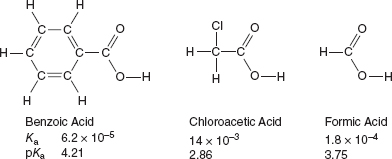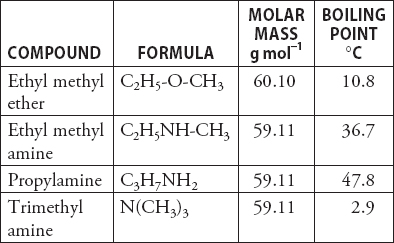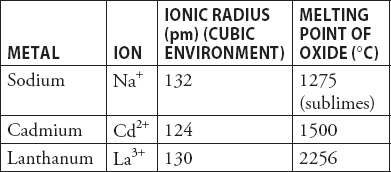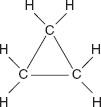# AP Chemistry Practice Test 40

### Test Information12 questions18 minutes

`Questions 1-2 refer to the following information.Use the information on the acids in the following diagram to answer questions.`

1. Sample solutions of each of the three acids were titrated with 0.10 M sodium hydroxide, NaOH. Each of the acid solutions had a concentration of 0.10 M. Which of the acid titrations had the highest pH at the endpoint?

2. A student prepares three buffer solutions. Each solution is 1.0 M in one of the acids in the table and 1.0 M in its corresponding sodium salt. Which of the solutions has the greatest buffer capacity with respect to added NaOH and why?

3. Hypochlorous acid is an unstable compound, and one of the decomposition products is chlorine gas, Cl2. The decomposition of the acid lowers its concentration over time. What effect will the decomposition of one-fourth of the acid have on the agreement between the endpoint of the titration and the equivalence point during a titration with standard sodium hydroxide?

4. Three 25.00 mL samples of approximately 0.10 M phenol, C6H5OH, Ka = 1.0 × 10-10 were removed from a container and placed in separate 250 mL beakers. The samples were titrated with standard potassium hydroxide, KOH, solution. Cresol red was the acid-base indicator used in the titration. The samples required 31.75, 32.38, and 41.75 mL to reach the endpoint. Which of the following might explain why one of the samples required significantly more base to reach the endpoint?

5. During the study of the reaction A → 2B, a chemist constructs several graphs. The graph of [A] versus time and the graph of ln [A] versus time both give a curved line; however, the graph of 1/[A] versus time and gives a straight line. This implies the rate law is

6. The photoelectron spectrum of carbon has three equally sized peaks. What peak is at the lowest energy?

7.According to the data in the table above, which of the compounds has the strongest intermolecular forces?

8.Each of the ions in the table form stable oxides (Na2O, CdO, and La2O3). Lanthanum oxide, La2O3, has a melting point significantly higher than that of the other oxides. Which of the following is the best explanation of why this is true?

9. Which of the following is the strongest Brønsted-Lowry acid?

10. During the investigation of a chemical reaction by a chemistry student, she was able to determine that the reaction was nonspontaneous at 1 atm and 298 K. However, she learned that cooling the system with dry ice (-78°C) caused the reaction to become spontaneous. Which of the following combinations must apply to this reaction?

11. What is the ionization constant, Ka, for a weak monoprotic acid if a 0.060 molar solution has a pH of 2.0?

12.Cyclopropane, pictured above, is a relatively unstable compound. As seen in the diagram, the carbon atoms form the corners of an equilateral triangle and each carbon atom has two hydrogen atoms attached to complete an octet of electrons around the carbon atoms. Based upon this structure, why is cyclopropane a relatively unstable compound?